Documentation

## Heat Distribution in Circular Cylindrical Rod

This example shows how to analyze a 3-D axisymmetric model by using a 2-D model.

The model geometry, material properties, and boundary conditions must all be symmetric about a single axis for this simplification from 3-D to 2-D to be appropriate. Because of this symmetry, a cylindrical coordinate system is the most convenient form for defining the partial differential equation. However, Partial Differential Equation Toolbox™ expects the equations in a Cartesian system. One of the main goals of this example is to show how to express the PDE defined in a cylindrical system in a Cartesian form that Partial Differential Equation Toolbox™ can handle.

This particular example shows heat transfer in a rod with a circular cross section. There is a heat source at the left end of the rod and a fixed temperature at the right end. The outer surface of the rod exchanges heat with the environment due to convection. In addition, heat is generated within the rod due to radioactive decay.

We would like to calculate the temperature in the rod as a function of time. The parabolic equation describing heat transfer is

`$\rho C\frac{\partial u}{\partial t}-\nabla \cdot \left(k\nabla u\right)=q,$`

where $\rho ,C,$ are the density, specific heat, and thermal conductivity of the material, respectively, $u$ is the temperature, and $q$ is the heat generated in the rod.

Since the problem is axisymmetric, it is convenient to write this equation in a cylindrical coordinate system.

`$\rho C\frac{\partial u}{\partial t}-\frac{1}{r}\frac{\partial }{\partial r}\left(kr\frac{\partial u}{\partial r}\right)-\frac{1}{{r}^{2}}\frac{\partial }{\partial \theta }\left(k\frac{\partial u}{\partial \theta }\right)-\frac{\partial }{\partial z}\left(k\frac{\partial u}{\partial z}\right)=q,$`

where $r,\theta$, and $z$ are the three coordinate variables of the cylindrical system. Because the problem is axisymmetric, $\partial u/\partial \theta =0$ and after multiplying by $r$ the equation can be rewritten

`$r\rho C\frac{\partial u}{\partial t}-\frac{\partial }{\partial r}\left(kr\frac{\partial u}{\partial r}\right)-\frac{\partial }{\partial z}\left(kr\frac{\partial u}{\partial z}\right)=rq.$`

The equation can be converted to the form supported by PDE Toolbox if $r$ is defined as $y$ and $z$ is defined as $x$. Rewriting the above equation gives

`$\rho Cy\frac{\partial u}{\partial t}-\nabla \cdot \left(ky\nabla u\right)=qy.$`

In transient problems of this type it is often useful to first compute the steady state solution-- the solution to the time-independent, elliptic equation. If the final time in the transient analysis is sufficiently large, the transient solution at the final time should be close to this steady state solution. This provides a valuable check on the accuracy of the transient analysis.

Create a thermal model for steady-state analysis.

`thermalModelS = createpde('thermal');`

The 2-D model is a rectangular strip whose y-dimension extends from the axis of symmetry to the outer surface and x-dimension extends over the actual length of the rod (from -1.5 m to 1.5 m). The geometry and mesh for this rectangular section are easily defined by specifying the x and y locations of the four corners as shown below.

`g = decsg([3 4 -1.5 1.5 1.5 -1.5 0 0 .2 .2]');`

Convert the geometry and append it to the thermal model.

`geometryFromEdges(thermalModelS,g);`

The rod is composed of a material with the following thermal properties.

```k = 40; % thermal conductivity, W/(m-degree C) rho = 7800; % density, kg/m^3 cp = 500; % specific heat, W-s/(kg-degree C) q = 20000; % heat source, W/m^3```

PDE Toolbox allows definition of the non-constant coefficients as function of spatial coordinates and solution.

```kFunc = @(region,state) k*region.y; cFunc = @(region,state) cp*region.y; qFunc = @(region,state) q*region.y;```

For a steady-state analysis, specify the thermal conductivity of the material.

`thermalProperties(thermalModelS,'ThermalConductivity',kFunc);`

Specify internal heat source.

`internalHeatSource(thermalModelS,qFunc);`

When defining boundary conditions below, it is necessary to know the edge numbers for the boundary edges of the geometry. A convenient way to obtain these edge numbers is to plot the geometry using `pdegplot` with option `edgeLabels` set to `'on'`.

```figure pdegplot(thermalModelS,'EdgeLabels','on'); axis equal xlim([-2 2]); title 'Rod Section Geometry With Edge Labels Displayed';```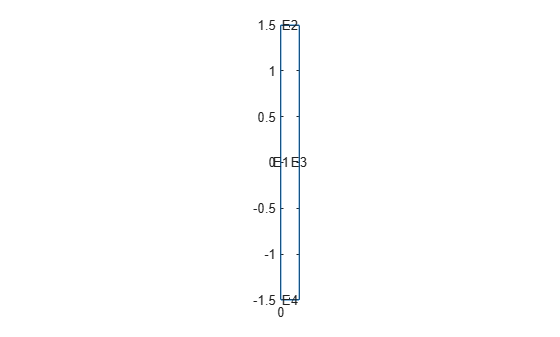Define the boundary conditions. Edge 1, which is the edge at $y$ equal zero, is along the axis of symmetry so there is no heat transferred in the direction normal to this edge. This boundary is modeled by the default as an insulated boundary. Edge 2 is kept at a constant temperature T = 100 C. Boundary conditions for the edges 3 and 4 are functions of `y`.

```thermalBC(thermalModelS,'Edge',2,'Temperature',100); outerCC = @(region,~) 50*region.y; thermalBC(thermalModelS,'Edge',3,... 'ConvectionCoefficient',outerCC,... 'AmbientTemperature',100); leftHF = @(region,~) 5000*region.y; thermalBC(thermalModelS,'Edge',4,'HeatFlux',leftHF);```

Generate the mesh.

```generateMesh(thermalModelS,'Hmax',0.1); figure; pdeplot(thermalModelS); axis equal title 'Rod Section With Triangular Element Mesh'```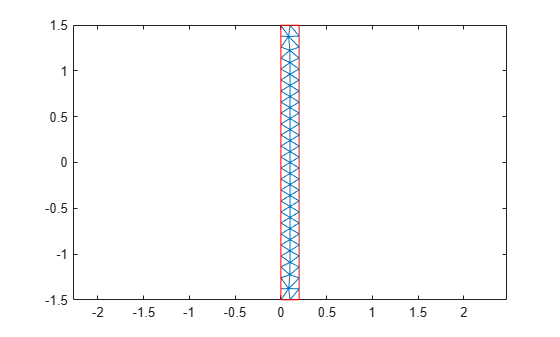Solve the model and plot the result.

```result = solve(thermalModelS); T = result.Temperature; figure; pdeplot(thermalModelS,'XYData',T,'Contour','on'); axis equal title 'Steady State Temperature';```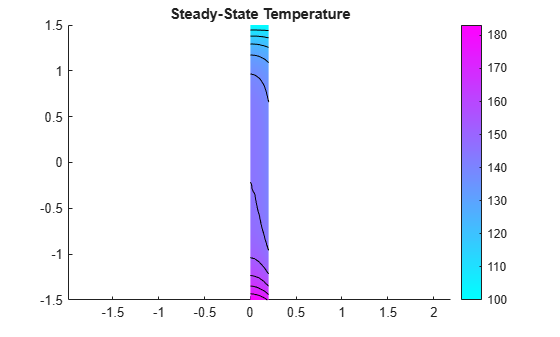### Transient Solution

Create a thermal model for transient analysis, include the geometry, and mesh.

```thermalModelT = createpde('thermal','transient'); g = decsg([3 4 -1.5 1.5 1.5 -1.5 0 0 .2 .2]'); geometryFromEdges(thermalModelT,g); generateMesh(thermalModelT,'Hmax',0.1);```

For a transient analysis, specify the thermal conductivity, mass density, specific heat of the material.

```thermalProperties(thermalModelT,'ThermalConductivity',kFunc,... 'MassDensity',rho,... 'SpecificHeat',cFunc);```

Specify internal heat source and boundary conditions.

```internalHeatSource(thermalModelT,qFunc); thermalBC(thermalModelT,'Edge',2,'Temperature',100); thermalBC(thermalModelT,'Edge',3,... 'ConvectionCoefficient',outerCC,... 'AmbientTemperature',100); thermalBC(thermalModelT,'Edge',4,'HeatFlux',leftHF);```

Compute the transient solution for solution times from $t=0$ to $t=20000$ seconds. Initial temperature in the rod is zero.

```tfinal = 20000; tlist = 0:100:tfinal; thermalIC(thermalModelT,0); thermalModelT.SolverOptions.ReportStatistics = 'on'; result = solve(thermalModelT,tlist);```
```114 successful steps 1 failed attempts 232 function evaluations 1 partial derivatives 22 LU decompositions 231 solutions of linear systems ```
`T = result.Temperature;`

Plot the solution at `t = 20000`.

```figure; pdeplot(thermalModelT,'XYData',T(:,end),'Contour','on'); axis equal title(sprintf('Transient Temperature at Final Time (%g seconds)',tfinal));```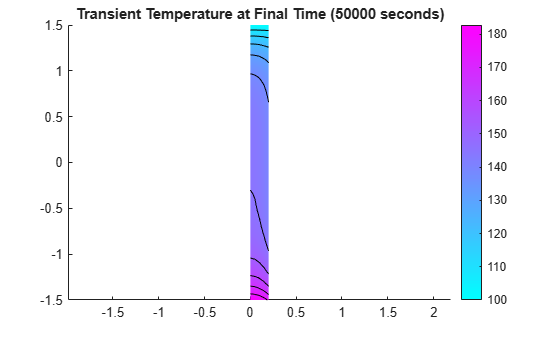The steady state solution and the transient solution at 20000 seconds are in close agreement. This can be seen by comparing the two figures.

The third figure below shows the temperature at the left end of the rod as a function of time. The outer surface of the rod is exposed to the environment with a constant temperature of 100 degrees-C. Consequently, when the surface temperature of the rod is less than 100, the rod is being heated by the environment and when greater than 100, cooled. When the rod temperature is less than 100 degrees, the outer surface is slightly warmer than the inner axis but when the temperature is around 100 degrees, the outer surface becomes cooler than the interior of the rod.

Find nodes on the left end of the rod and on the center axis and outer surface using their coordinate values.

```p = thermalModelT.Mesh.Nodes; nodesLeftEnd = find(p(1,:) < -1.5+eps); nodeCenter = nodesLeftEnd(p(2,nodesLeftEnd) < eps); nodeOuter = nodesLeftEnd(p(2,nodesLeftEnd) > 0.2-eps); figure; plot(tlist,T(nodeCenter,:)); hold all plot(tlist,T(nodeOuter,:),'--'); title 'Temperature at Left End as a Function of Time' xlabel 'Time, seconds' ylabel 'Temperature, degrees-C' grid on; legend('Center Axis','Outer Surface','Location','SouthEast');```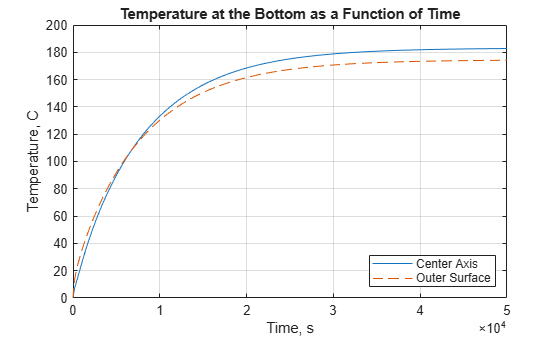##### Support평가판 신청